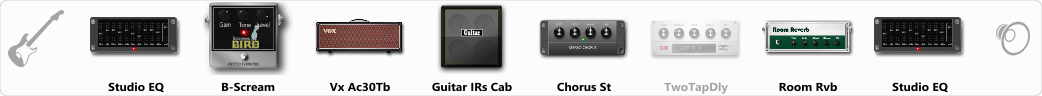# Brian May inspired

Discussion in 'ToneLib-GFX presets' started by Aslam, Jul 21, 2019.

1. Brian May inspired

Preset name: Bri

created while using an epiphone les paul custom pro - works well with humbuckers and single-coils

Effects chain:Effect: "Studio EQ" (Dynamics / Filter), active - "yes"
"31 Hz" = -1
"62 Hz" = -1
"125 Hz" = 0
"250 Hz" = 0
"500 Hz" = 0
"1 kHz" = 9
"2 kHz" = 3
"4 kHz" = 3
"8 kHz" = 5
"16 kHz" = 3
"above 16 kHz" = 0
"Level (dB)" = 0

Effect: "B-Scream" (Overdrive / Distortion), active - "yes"
"Drive" = 0
"Tone" = 100
"Level" = 100

Effect: "Vx Ac30Tb" (Amp simulators), active - "yes"
"Gain" = 100
"Bass" = 25
"Middle" = 25
"Treble" = 25
"Presence" = 25
"Master" = 100
"Output" = 50
"Level (dB)" = 0

Effect: "Guitar IRs Cab" (Cabinets), active - "yes"
"Model" = Vox AC30 (2x12")
"Mic Position" = Center
"Mic Distance" = Middle
"Low Cut (Hz)" = 60
"Hi Cut (kHz)" = 20.0
"Mix" = 100
"Level (dB)" = 0

Effect: "Chorus St" (Modulation / Sfx), active - "yes"
"Speed" = 1.2
"Depth" = 38
"Center" = 5.0
"Mix" = 42

Effect: "TwoTapDly" (Delay), active - "no"
"Time" = 1600
"Feedback" = 21
"Tone" = 48
"Sens" = 67
"TapTime" = 5.0
"Mix" = 59

Effect: "Room Rvb" (Reverb), active - "yes"
"Time" = 4.1
"PreDelay" = 4
"LoDamp" = 32
"HiDamp" = 38
"Mix" = 67

Effect: "Studio EQ" (Dynamics / Filter), active - "yes"
"31 Hz" = 0
"62 Hz" = 0
"125 Hz" = 0
"250 Hz" = 0
"500 Hz" = 0
"1 kHz" = 0
"2 kHz" = 0
"4 kHz" = 0
"8 kHz" = 0
"16 kHz" = 0
"above 16 kHz" = 0
"Level (dB)" = -2

Note: You will need to download and install the ToneLib-GFX software to use the preset.

File size:
764 bytes
Views:
8,389